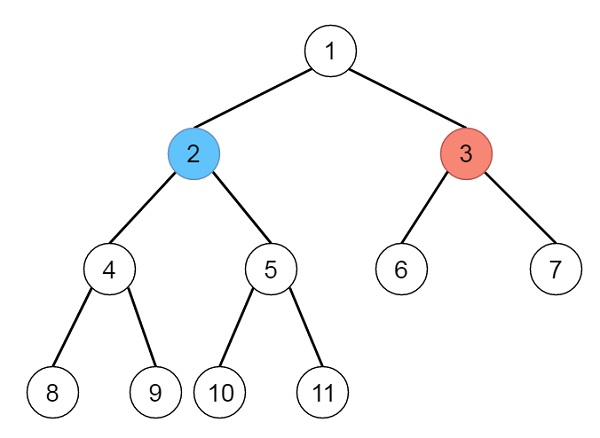# Binary Tree Coloring Game in Python

Suppose there are two players play a turn-based game on a binary tree. We have the root of this binary tree and the number of nodes n in the tree. Here n is odd, and each node has a distinct value from 1 to n. At first, the first player names a value x with 1 <= x <= n, and the second player names a value y with 1 <= y <= n and that holds a condition, such that y != x. The first player colors the node with value x red, and the second player colors the node with value y blue. After that, the players take turns starting with the first player. In each turn, player takes a node of their color (red for player 1, blue for player 2) and colors an uncolored neighbor of the chosen node (either left or right child, or parent of the taken node.). If and only if a player cannot take such a node in this way, they must pass their turn. If both players pass their turn, the game will be ended, and the winner is the player that colored more nodes.

Suppose we are the second player. If it is possible to choose such a y to ensure we win the game, return true. If it is not possible, return false.

So if the tree is like −and n is 11 and x is 3, then the output will be true, as the second player can take the node with value 2

To solve this, we will follow these steps −

• Define a method called solve(), this will take node, x, l and r, the l and r are initially false, this will act like below −

• if node is not present, then return and exit

• if l is true, then increase leftVal by 1, otherwise when r is true, then increase rightVal by 1

• if node value is x, then call solve(left of node, x, true, false) and call solve(right of node, x, false, true)

• otherwise call solve(left of node, x, l, r) and call solve(right of node, x, l, r)

• The main method will be like −

• nodeToX := 0, leftVal := 0, rightVal := 0

• call solve(root, x, false, false)

• nodeToX := n – leftVal – rightVal – 1

• temp := maximum of rightVal, nodeToX and leftVal

• return false if (nodeToX + leftVal + rightVal – (2*temp) >= 0), otherwise true

## Example(Python)

Let us see the following implementation to get a better understanding −

Live Demo

class TreeNode:
def __init__(self, data, left = None, right = None):
self.data = data
self.left = left
self.right = right
def insert(temp,data):
que = []
que.append(temp)
while (len(que)):
temp = que
que.pop(0)
if (not temp.left):
if data is not None:
temp.left = TreeNode(data)
else:
temp.left = TreeNode(0)
break
else:
que.append(temp.left)
if (not temp.right):
if data is not None:
temp.right = TreeNode(data)
else:
temp.right = TreeNode(0)
break
else:
que.append(temp.right)
def make_tree(elements):
Tree = TreeNode(elements)
for element in elements[1:]:
insert(Tree, element)
return Tree
class Solution(object):
def btreeGameWinningMove(self, root, n, x):
self.nodeToX = 0
self.leftVal = 0
self.rightVal = 0
self.solve(root,x)
self.nodeToX = n - self.leftVal - self.rightVal - 1
temp = max(self.rightVal,max(self.nodeToX,self.leftVal))
return not (self.nodeToX + self.leftVal + self.rightVal - (2*temp)>=0)
def solve(self,node,x,l= False,r = False):
if not node:
return
if l:
self.leftVal+=1
elif r:
self.rightVal+=1
if node.data == x:
self.solve(node.left,x,True,False)
self.solve(node.right,x,False,True)
else:
self.solve(node.left,x,l,r)
self.solve(node.right,x,l,r)
ob = Solution()
root = make_tree([1,2,3,4,5,6,7,8,9,10,11])
print(ob.btreeGameWinningMove(root, 11, 3))

## Input

[1,2,3,4,5,6,7,8,9,10,11]
11
3

## Output

true